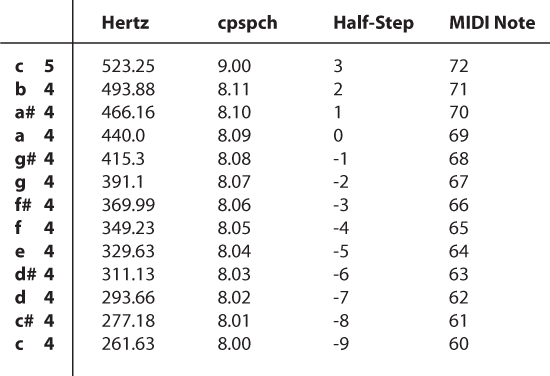# Four ways for doing Twelve-tone Equal TemperamentAs a general rule, Csound unit generators require that frequency is specified in terms of hertz, cycles per second. Though playing a middle C by typing a value of 261.63 is both unintuitive and impractical. There are several ways of tackling this issue within in Csound, and I’ll step you through a few in this example.

The easiest way for doing twelve-tone equal temperament in Csound is to utilize the cpspch opcode, which translates a value in octave point pitch-class notation (8ve.pc) to hertz. The value for A440 in 8ve.pc is represented as 8.09. The 8 represents the octave, while the 09 is the number of half steps above the C for the specified octave. Middle C is represented as 8.00.

Another way of doing this is by specifying a formula ourselves in the code. With instr 3, users can choose a pitch by specifying the number of half-steps away from A440. For example, if p4 equals 0, the instr will sound a pitch of 440Hz. A value of -9 will sound middle C.

MIDI is a well established format that has its own way of doing things. Instrument 4 uses a modified version of instr 3’s formula, so that it accepts MIDI note value. Middle C in MIDI is 60, and entering this value will in fact sound middle C.

Here are the four formulas used in the example csd:

```ifreq = p4 ; Hertz ipch = cpspch(p4) ; cpspch ihalf_step = 440 * 2 ^ (p4 / 12) ; Half-step (relative to A440) imidi_note = 440 * 2 ^ ((p4 - 69) / 12) ; MIDI note```

Synthesis Fall 2010

Update: Steven Yi, creator of Blue (a music composition environment for Csound) and co-editor of The Csound Journal, correctly points out that cps2pch is more accurate for tuning than cpspch. Definitely worth knowing.

## One thought on “Four ways for doing Twelve-tone Equal Temperament”

1. Justa note, cpspch can be slightly inaccurate due to using a lookup table (mentioned in the manual). cpspch is faster, but using cps2pch is more accurate.